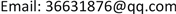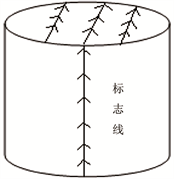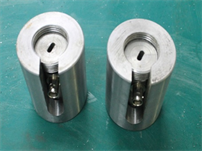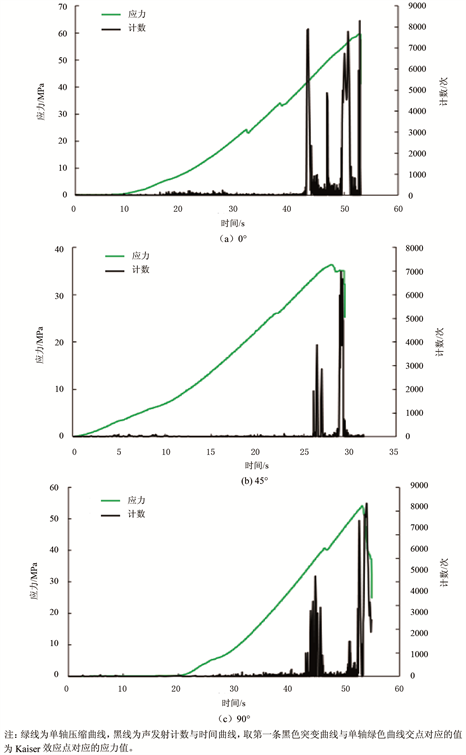﻿ 声发射与古地磁结合确定天然裂缝方向 Determining the Direction of Natural Fractures by Combining Acoustic Emission with Paleomagnetism

Journal of Oil and Gas Technology
Vol.40 No.05(2018), Article ID:27423,6 pages
10.12677/JOGT.2018.405103

Determining the Direction of Natural Fractures by Combining Acoustic Emission with Paleomagnetism

Wei Li1, Xiaowei Gong2, Kelin Wang3, Jin Zhou2

1School of Petroleum Engineering, Northeast Petroleum University, Daqing Heilongjiang

2Exploration Division, Tarim Oilfield Company, PetroChina, Korla Xinjiang

3Oil and Gas Engineering Research Institute, Tarim Oilfield Company, PetroChina, Korla XinjiangReceived: Sep. 27th, 2017; accepted: Apr. 20th, 2018; published: Oct. 15th, 2018ABSTRACT

The application of hydraulic fracturing in the oilfields was more and more extensive, and the existence of natural fractures in the reservoir affected the extension direction of the hydraulic fractures. Therefore, the accurate description of the natural fractures in the reservoirs was of vital importance for determining the fracture morphology in hydraulic fracturing, the ability to generate complex fractures as well as the direction of well array and well pattern selection. Therefore, by combining acoustic emission with paleomagnetic experiments, according to the acoustic emission experiment, the direction of the marker line relative to the earth stress was obtained, and the direction of the rock core mark line relative to the modern geographical north pole was determined by the paleomagnetic method. The direction of natural fractures in Fuyu Reservoir in the south of Changyuan Area in Daqing Oilfield was determined. According to the acoustic emission experiment, the direction of the mark line relative to the geostress is obtained. The paleomagnetic method is used to determine the orientation of the core mark line relative to the modern geography. The results show that the direction of the natural fractures obtained by combining the acoustic emission and paleomagnetism is close to that of formation dip logging curve.

Keywords:Hydraulic Fracturing, Natural Fracture, Acoustic Emission, Paleomagnetism, In-situ Stress Direction

1东北石油大学石油工程学院，黑龙江 大庆

2中石油塔里木油田分公司勘探事业部，新疆 库尔勒

3中石油塔里木油田分公司油气工程研究院，新疆 库尔勒1. 前言

2. 基本原理

3. 试验岩样的制备Figure 1. The preparation of acoustic emission and paleomagnetic rock samplesFigure 2. The acoustic emission probe specimen

4. 数据处理方法Figure 3. The relationship of stress and count changing with time of rock core specimen in different directions

${\sigma }_{\theta }={\sigma }_{x}{\mathrm{cos}}^{2}\theta +{\sigma }_{y}{\mathrm{sin}}^{2}\theta +2{\tau }_{xy}\mathrm{sin}\theta \mathrm{cos}\theta$ (1)

${\sigma }_{\text{H}}=\frac{{\sigma }_{x}+{\sigma }_{y}}{2}+{\left[\frac{{\left({\sigma }_{x}-{\sigma }_{y}\right)}^{2}}{4}+{\tau }_{xy}^{2}\right]}^{\frac{1}{2}}$ (2)

${\sigma }_{\text{h}}=\frac{{\sigma }_{x}+{\sigma }_{y}}{2}-{\left[\frac{{\left({\sigma }_{x}-{\sigma }_{y}\right)}^{2}}{4}+{\tau }_{xy}^{2}\right]}^{\frac{1}{2}}$ (3)

$\alpha =\frac{1}{2}{\mathrm{tan}}^{-1}\left(\frac{2{\tau }_{xy}}{{\sigma }_{x}-{\sigma }_{y}}\right)$ (4)

$\text{tg}I=2\text{tg}L$ (5)

5. 试验结果Table 1. The results of acoustic emission and paleomagnetic measurementsTable 2. The main stress measurement results of acoustic emission and paleomagnetism

6. 结语

 邓虎成, 周文, 周秋媚, 等. 新场气田须二气藏天然裂缝有效性定量表征方法及应用[J]. 岩石学报, 2013, 29(3): 1087-1097.

 李志明, 张金珠. 地应力与油气勘探开发[M]. 北京: 石油工业出版社, 1997: 109.

 陈强, 朱宝龙, 胡厚田. 岩石Kaiser效应测地应力场的试验研究[J]. 岩石力学与工程学报, 2006, 25(7): 1370-1376.

 张广清, 金衍, 陈勉. 利用围压下岩石的凯泽效应测定地应力[J]. 岩石力学与工程学报, 2002, 21(3): 360-363.

 杨杰. 声发射信号处理与分析技术的研究[D]: [硕士学位论文]. 长春: 吉林大学, 2005.

 侯守信, 田国荣. 古地磁岩心定向及其在地应力测量上的应用[J]. 地质力学学报, 1999, 5(1): 92-98.

 侯守信, 田国荣. 粘滞剩磁(VRM)岩芯定向的应用[J]. 岩石力学与工程学报, 2000, 19(S1): 1128-1131.

 黄宝春, 谭承泽. 古地磁多磁成分的分离技术[J]. 地球物理学进展, 1994, 9(1): 1128-1131.

 李素玲, 程国良, 孙宇航. 古地磁法在钻孔岩芯定位和隐伏断裂研究中的应用[J]. 地球物理学进展, 1993, 8(4): 262-263.

 李彦兴, 董平川. 利用岩石的Kaiser效应测定储层地应力[J]. 岩石力学与工程学报, 2009, 28(S1): 2802-2807.

 田国荣. 差应变分析与古地磁结合确定地应力方向[D]: [硕士学位论文]. 北京: 中国地质大学(北京), 2003.

[编辑] 龚丹